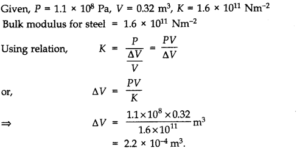# Chapter 9 – Mechanical Properties Of Solids Questions and Answers: NCERT Solutions for Class 11 Physics

Class 11 Physics NCERT book solutions for Chapter 9 - Mechanical Properties Of Solids Questions and Answers.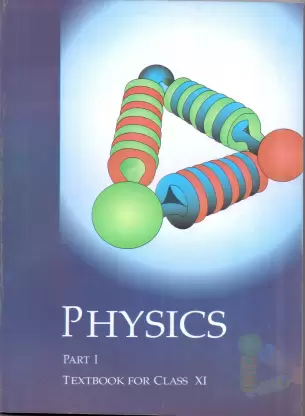## Question 9. 1. A steel wire of length 4.7 m and cross-sectional area 3.0 x 10-5m2stretches by the same amount as a copper wire of length 3.5 m and cross-sectional area of 4.0 x 10-5m2under a given load. What is the ratio of the Young’s modulus of steel to that of copper?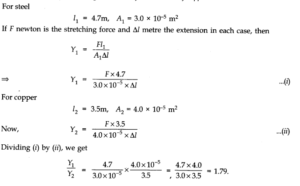## Question 9. 2. Figure shows the strain-stress curve for a given material. What are (a) Young’s modulus and (b) approximate yield strength for this material?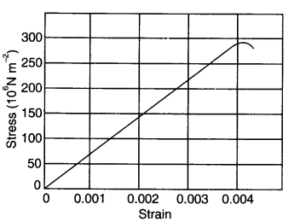## Question 9. 3. The stress-strain graphs for materials A and B are shown in figure.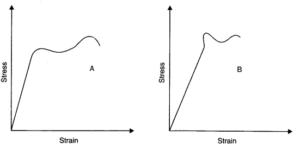## Question 9. 5. Two wires of diameter 0.25 cm, one made of steel and other made of brass are loaded as shown in figure. The unloaded length of steel wire is 1.5 m and that of brass wire is 1.0 m.Young’s modulus of steel is 2.0 x 1011Pa. Compute the elongations of steel and brass wires. (1 Pa = 1 Nm2).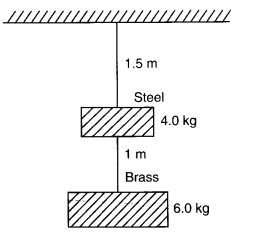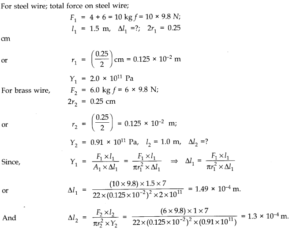## Question 9. 6. The edge of an aluminium cube is 10 cm long. One face of the cube is firmly fixed to a vertical wall. A mass of 100 kg is then attached to the opposite face of the cube. The shear modulus of aluminium is 25 GPa. What is the vertical deflection of this face?

### Answer:Here, side of cube, L = 10 cm =10/100= 0.1 m .•. Area of each face, A = (0.1)2= 0.01 m2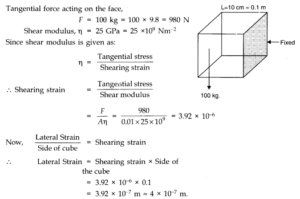## Question 9. 7. Four identical hollow cylindrical columns of mild steel support a big structure of mass 50,000 kg. The inner and outer radii of each column are 30 cm and 60 cm respectively. Assuming the load distribution to be uniform, calculate the compressional strain of each column. Young’s modulus, Y = 2.0 x 1011Pa.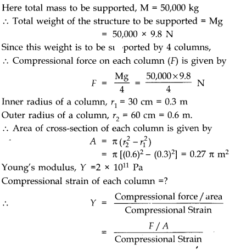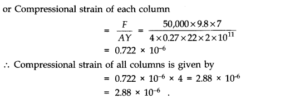## Question 9. 8. A piece of copper having a rectangular cross-section of 15.2 mm x 19.1 mm is pulled in tension with 44,500 N force, producing only elastic deformation. Calculate the resulting strain? Shear modulus of elasticity of copper is 42 x 109N/m2.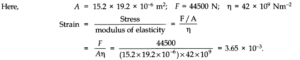## Question 9. 9. A steel cable with a radius of 1.5 cm supports a chairlift at a ski area. If the maximum stress is not to exceed 108Nm-2what is the maximum load the cable can support ?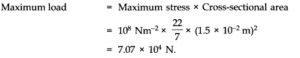## Question 9. 10. A rigid bar of mass 15 kg is supported symmetrically by three wires each 2.0 m long. Those at each end are of copper and the middle one is of iron. Determine the ratios of their diameters if each is to have the same tension.

### Answer:Since each wire is to have same tension therefore, each wire has same extension. Moreover, each wire has the same initial length.So, strain is same for each wire.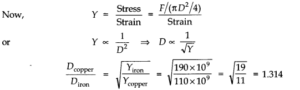## Question 9. 11. A 14.5 kg mass, fastened to the end of a steel wire of unstretched length 1 m, is whirled in a vertical circle with an angular velocity of 2 rero./s at the bottom of the circle. The cross-sectional area of the wire is 0.065 cm2. Calculate the elongation of the zvire when the mass is at the lowest point of its path. Ysteel = 2 x 1011Nm-2.

### Ans.Here, m = 14.5 kg; l = r = 1 m; v = 2 rps; A = 0.065 x 10-4m2Total pulling force on mass, when it is at the lowest position of the vertical circle is F = mg + mr w2= mg + mr 4,π2v2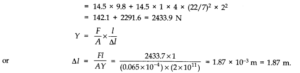## 9.12.Compute the bulk modulus of water from the following data: Initial volume = 100.0 litre, Pressure increase = 100.0 atm (1 atm = 1.013 x 105Pa), Final volume = 100.5 litre. Compare the bulk modulus of water with that of air (at constant temperature). Explain in simple terms why the ratio is so large.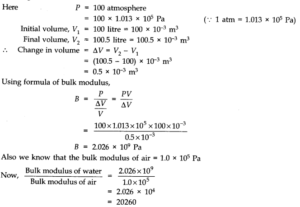## Question 9. 13. What is the density of water at a depth where pressure is 80.0 atm, given that its density at the surface is 1.03 x 103kg m-3?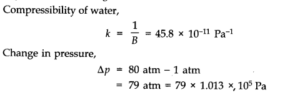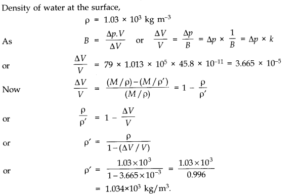## Question 9. 14. Compute the fractional change in volume of a glass slab, when subjected to a hydraulic pressure of 10 atm.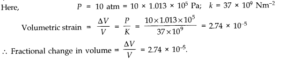## Question 9. 15. Determine the volume contraction of a solid copper cube, 10 cm on an edge, when subjected to a hydraulic pressure of 7.0 x 106Pa.

### Answer:Here a side of copper cube a = 10 cm, hence volume V = a3= 10-3 m3, hydraulic pressure applied p = 7.0 x 106Pa and from table we find that bulk modulus of copper B = 140 G Pa = 140 x 109Pa.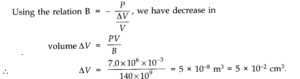## Question 9. 16. How much should be pressure the a litre of water be changed to compress it by 0.10 %? Bulk modulus of elasticity of water = 2.2 x 109Nm-2.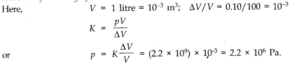## Question 9. 17. Anvils made of single crystals of diamond, with the shape as shown in figure are used to investigate behaviour of materials under very high pressures. Flat faces at the narrow end of the anvil have a diameter of 0.50 mm, and the wide ends are subjected to a compressional force of50,000 N. What is the pressure at the tip of the anvil?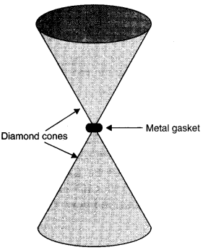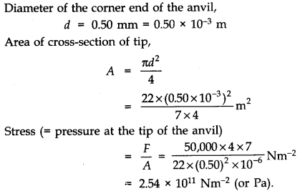## Question 9. 18. A rod of length 1.05 m having negligible mass is supported at its ends by two wires of steel (wire A ) and aluminium (wire B) of equal lengths as shown in figure.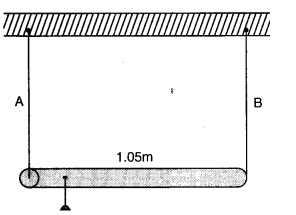## The cross-sectional areas of wires A and B are 1.0 mm2and 2.0 mm2, respectively. At what point along the rod should a mass m be suspended in order to produce (a) equal stresses and (b) equal strains in both steel and aluminium wires.

### Answer:For steel wire A, l1=l; Az= 1 mm2; Y1= 2 x 1011 Nm-2 For aluminium wire B, l2= l; A2= 2mm2; Y2= 7 x 1010 Nm-2 (a) Let mass m be suspended from the rod at distance x from the end where wire A is connected. Let F1and F2be the tensions in two wires and there is equal stress in two wires, then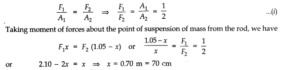### (b) Let mass m be suspended from the rod at distance x from the end where wire A is connected. Let F1and F2be the tension in the wires and there is equal strain in the two wires i.e.,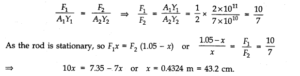## Question 9. 19. A mild steel wire of length 1.0 m and cross-sectional area 0.50 x 10-2cm2is stretched, well within its elastic limit, horizontally between two pillars. A mass of 100g is suspended from the mid-point of the wire. Calculate the depression at the mid-point.

### Answer:Let AB be a mild steel wire of length 2L = lm and its cross-section area A = 0.50 x 10-2cm2. A mass m = 100 g = 0.1 kg is suspended at mid-point C of wire as shown in figure. Let x be the depression at mid-point i.e., CD = x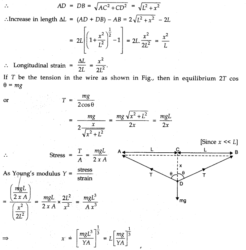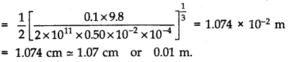## Question 9. 21. The Marina trench is located in the Pacific Ocean, and at one place it is nearly eleven km beneath the surface of water. The water pressure at the bottom of the trench is about 1.1 x 108Pa. A steel ball of initial volume 0.32 m3is dropped into the ocean and falls to the bottom of the trench. What is the change in the volume of the ball when it reaches to the bottom?